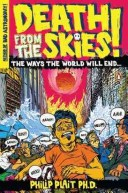### Ray representations and Galilei group

For my PhD thesis I performed a work in group teory, precisely in the theory of representations, applied to quantum mechanics. So, in order to describe my work, recently published by the Journal of Mathematical Physics, I need to introduce some concepts. The group theory was founded indipendetly by Niels Abel and Evariste Galois and it is focused on group, a set $G$ of elements with a multiplication operation $\cdot$ and such that the following properties are true(9):
1. $\forall a, b \in G, a \cdot b \in G$
2. $\forall a, b, c \in G, a(bc) = (ab) c$
3. $\forall a \in G \, \exists e \in G \, \text{:} \, ae = ea = a$
4. $\forall a \in G \, \exists b \in G \, \text{:} \, ab = ba = e$ and $b = a^{-1}$
A group is called abelian group if
1. $\forall a, b \in G, ab = ba$
For every group, we can write a representation, that is a set of operators or functions that act in a mathematical space and change it in a same way at the elements of the group change their own space(1). In other (trivial) words we have a given world with a group (for example our real world with a group of symmetry, for example the rotations), and in order to find properties of this world we must use a mathematical representation.
If the world is a given physical system (for example a free particle), we have a symmetry group, that is a set of all symmetry transformation(2) of our physical system, and his representation acts in a so called Hilbert space. In this space, following Wigner's theorem(4), the most general representation is a ray (unitary) representation. In order to understand the ray (or projective) representations, we must enunciate the theorem:
For every transformation of symmetry $T: \mathcal R \rightarrow \mathcal R$ between the rays of a Hilbert space $\mathcal H$ and such that conserve the transition probabilities, we can define an operator $U$ on the Hilbert space $\mathcal H$ such that, if $|\psi> \in {\mathcal R}_\psi$, then $U |\psi> \in {\mathcal R}'_\psi$, where ${\mathcal R}_\psi$ is the radius of the state $|\psi>$, ${\mathcal R}'_\psi = T {\mathcal R}_\psi$, and $U$ uniform and linear $< U \psi | U \varphi> = <\psi | \varphi>, \qquad U |\alpha \psi + \beta \varphi> = \alpha U |\psi> + \beta U |\varphi>$ or with $U$ antiunitario and antilinear: $< U \psi | U \varphi> = <\varphi | \psi>, \qquad U |\alpha \psi + \beta \varphi> = \alpha^* U |\psi> + \beta^* U |\varphi>$ Further, $U$ is uniquely determined except for a phase factor.
So a ray representation is the association between an element of the symmetry group $G$ to a set of unitary (or antiunitary) operators which differ only for a phase: in other worlds a ray of operators(3).

### Review: Death from the skies

#SciDoom
The universe is trying to kill you.
Philip Plait, Death from the skies
The Universe is the most dangerous place that you can imagine. There are a lot of perils: asteroids and comets, supernavae, gamma ray bursts and finally our star, the Sun. Every source of danger is examined in nine chapters introduced by a fictional short story, that is scientifically correct. In these introductory stories, Plait describes a possible scenario in which Earth is shotted by, for example, a comet o a great asteroid, like the one that leaves the Meteor Crater in Arizona(Commons)
or the ones that probably caused the dinosaurs extintion.In order to prevent a bad encounter with a great object, physicists describe a lot of possible solutions: in the list there are a nuclear bomb, an impact against an artificial object (like Deep Impact with Tempel 1) or try to change the bullet's trajectory using the gravitational force of an ather astetoids. This last solution is proposed by B612 Foundation and, like the others, present a lot of difficulties, but is technically realizable now!
Some possible problems are, for example with shot solutions, that the object is broken into too big pieces, while for the gravitational solution we must demonstrate a very precise control of the gravity. These are the only enemies we can prevent, but against the others we could realize some particular protection, like radiations from Sun or from supernovae explosions. In this last case, the probability to be shot is 1/10000000, against 1/700000 of an asteroid shot, that is a probability actually higher than terrorism!
Another danger from outer space is an... alien attack! Don't worry! I don't mean the little green men from Mars, but simply eventually microscopic life present in asteroids or comets. An attach like this has a very low probability, first of all because the aliens must survive at Earth atmosphere, and after because they have a low probability to interact with DNA developed in our environment: following our knowledge, we must remeber that in the Universe our planet is unique!
I don't know if we are alone or not, but in this moment it is so...
In the last part of the post I spend some words about Sun: